## Example Questions

### Example Question #7 : Monomials

Choose the answer that is the best solution to the following expression of monomial quotients: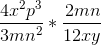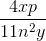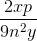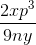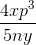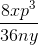Explanation:To multiply monomial quotients, treat them as you would any other fraction. Combine like terms wherever possible: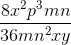Then, you need to reduce:### Example Question #8 : Monomials

Choose the answer that is the simplest form of the following expression of monomial quotients: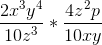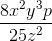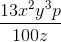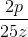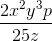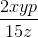Explanation:To simplify, first multiply across: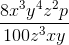Then, reduce: